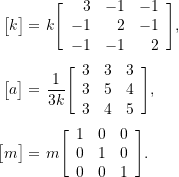### Approximate Methods for Multiple Degree of Freedom Systems: Dunkerley’s Formula

Dunkerley’s Formula is another method of estimating the lowest (fundamental) natural frequency of a system without having to solve an eigenvalue problem. Rather than using the stiffness matrix, Dunkerley’s Method makes use of the flexibility matrix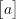which is the inverse of the stiffness matrix.

Starting with the equations of motion for a general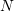degree of freedom system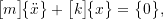and assuming simple simultaneous harmonic motion of the form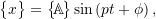results in

(9.9)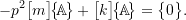Premultiplication of (9.9) by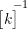results in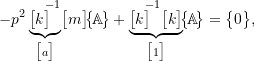so that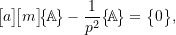or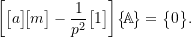As we have seen previously, for a non-trivial solution to exist it is required that

(9.10)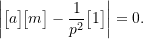For simplicity we will assume that the mass matrix is diagonal. In that case, the determinant in (9.10) has the form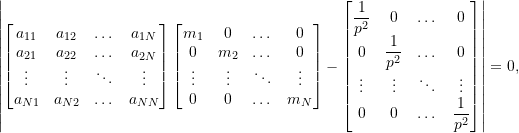or

(9.11)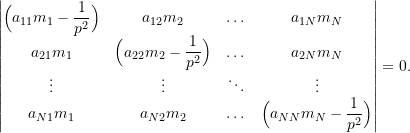Expanding (9.11) produces the characteristic equation which has the form

(9.12)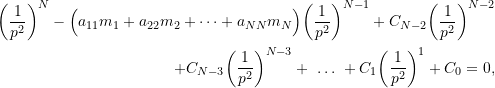where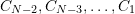and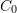are constants (which will depend onand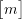) which we do not need to consider here.

Let theroots of (9.12) be given by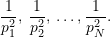Since thesevalues are the roots, the characteristic equation could also be written in the form

(9.13)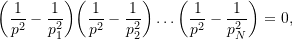which is a completely equivalent polynomial to (9.12). Expanding (9.13) we get

(9.14)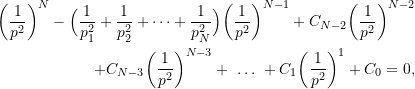whereandare the same constants which appeared in (9.12). Comparing the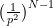terms in equations (9.12) and (\label{eq:Dunkerley_Char_II}) shows that

(9.15)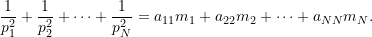To this point no approximations have been made. Now however, we note that for many systems the higher natural frequencies are significantly larger than the fundamental natural frequency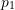so that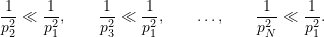As a result, ignoring all but the first term on the LHS of (9.15) results in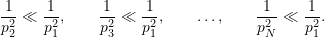As a result, ignoring all but the first term on the LHS of equation (9.15) results in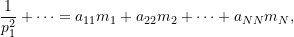or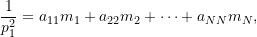or more generally

(9.16)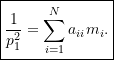Equation (9.16) is known as Dunkerley’s Formula and provides an estimate of the fundamental natural frequency of a system. Due to the terms that have been neglected, the natural frequency obtained using Equation (9.16) will be lower than the actual fundamental natural frequency. Dunkerley’s Formula therefore provides a lower bound on the lowest natural frequency of the system (in contrast to Rayleigh’s Quotient which provided an upper bound on the lowest natural frequency).

Note that as long as the mass matrix is diagonal, Equation (9.16)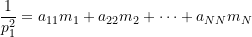can be written as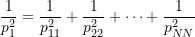where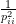is defined as the natural frequency of the single degree of freedom system that results when mass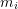is the only mass in the system (i.e. all other masses in the system are assumed to be zero).
This is because of the fact that ifis the only mass in the system then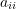represents the deflection of the mass (in the positive i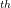coordinate direction) when a unit load is applied to the mass(again in the positive icoordinate direction). The effective stiffness of the (single degree of freedom) system is then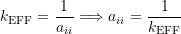so that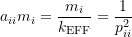as defined above. This interpretation can be useful in applying Dunkerley’s Formula in some instances.

### Example 1

Estimate the fundamental natural frequency for the system shown below using Dunkerley’s Method.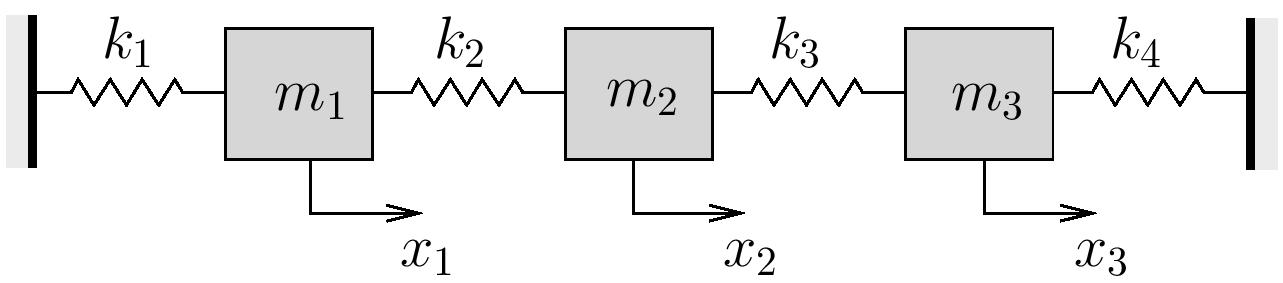Consider the following cases:

i)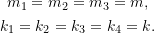ii)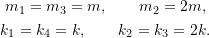iii)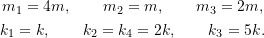Note that the stiffness matrix for this problem is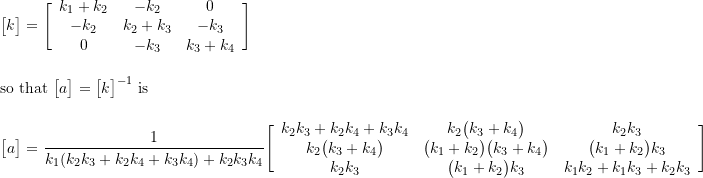### Example 2

Use Dunkerley’s method to estimate the fundamental natural frequency for a light simply supported beam supporting three equally spaced identical masses.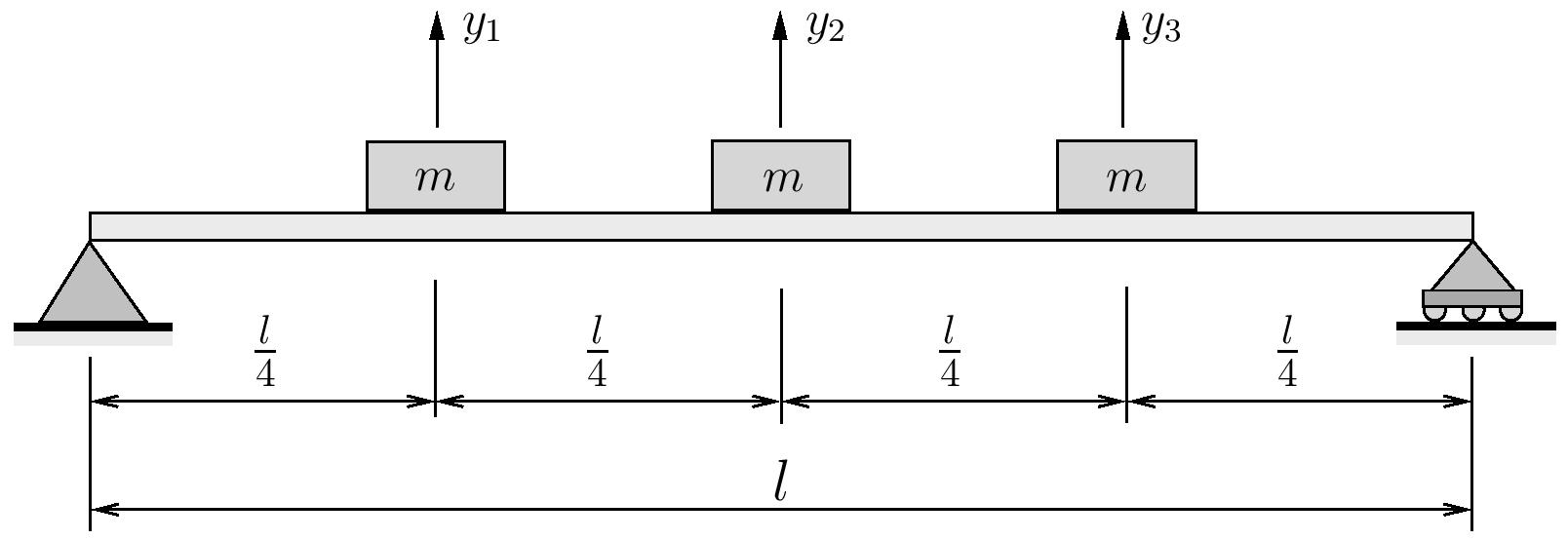Note that for this system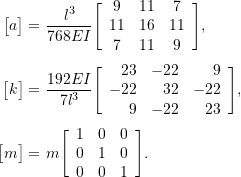### Dunkerley’s Formula to Estimate The Highest Natural Frequency

The ideas behind Dunkerley’s Formula, with a slightly different formulation, can also be used to estimate the highest natural frequency in a system. To see this start with (9.9)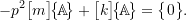Premultiplication by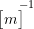produces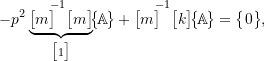or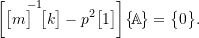This again requires, for non-trivial solutions,

(9.17)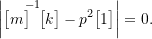If we again assume that the mass matrix is diagonal, then the inverse is simply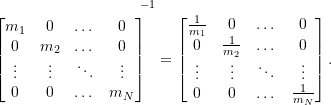If we again assume that the mass matrix is diagonal, then the inverse is simplyAs a result, the determinant in (9.17) has the form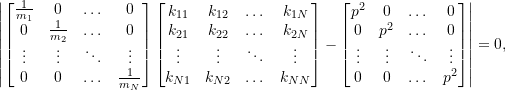or

(9.18)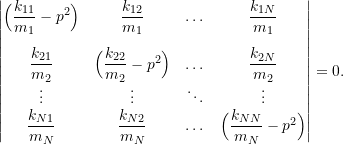Expanding (9.18) produces a characteristic equation with the form

(9.19)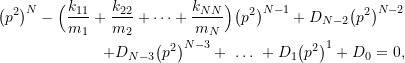where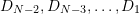and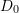are again constants which depend onand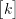.
Theroots of (9.19) correspond to the natural frequencies of the system given by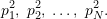Once again, since thesevalues are the roots of the characteristic equation, we could equivalently write the characteristic equation as

(9.20)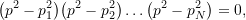which expanded gives

(9.21)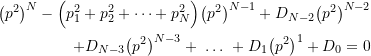whereandare the same constants as in (9.19) Comparing the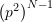terms in equations (9.19) and (9.21) shows that

(9.22)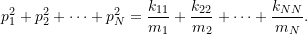Since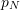is the largest natural frequency in the system, we ignore all of the other terms on the LHS of (9.22) to get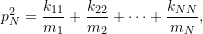or more generally

(9.23)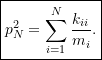Due to the terms that have been ignored in (9.22), the frequency obtained using (9.23) will be larger than the highest natural frequency in the system. As a result, this version of Dunkerley’s Formula provides an upper bound on the largest natural frequency of the system.

### Example

For the system shown below: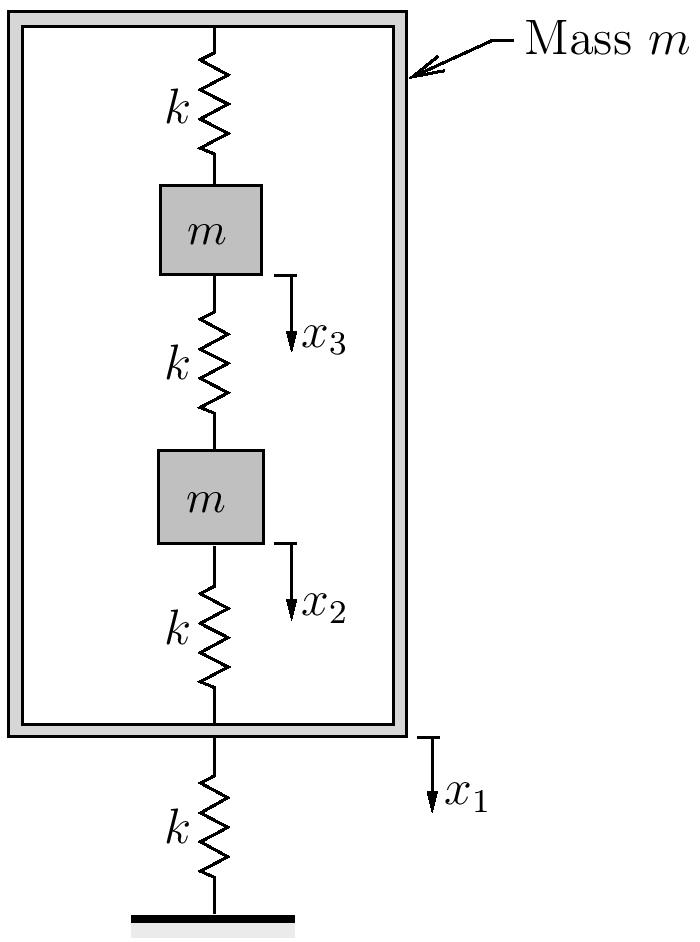1. Use matrix iteration to find the lowest and highest natural frequencies and associated mode shapes.
2. Use Rayleigh’s quotient to find an upper bound to the lowest natural frequency. Use a trial vector of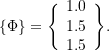3. Use Dunkerley’s method to find a lower bound for the lowest natural frequency.
4. Use Dunkerley’s method to find an upper bound for the highest natural frequency.

Note that for this problem,